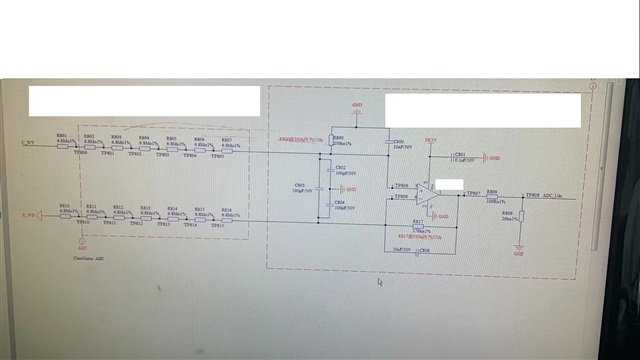If you have a related question, please click the "Ask a related question" button in the top right corner. The newly created question will be automatically linked to this question.

# OPA335: larger error than TLV314

Part Number: OPA335
Other Parts Discussed in Thread: TLV314,

Hi,

I'm using the circuit below to sense the DC BUS voltage, ADC_Udc=(135/23919)*Udc, ADC_Udc is fed to the ADC of MCU.

The DC bus voltage ranges from 50V to 500V.I compare the actual DC bus voltage and the DC bus voltage calculated from ADC results, the difference value is the error.

When I use TLV314 in the circuit, the error is 0.09V at 50V DC BUS, and 0.44V at 500V DC BUS.

When I use OPA335 in the circuit, the error is 0.25V at 50V DC BUS, and 2.03V at 500V DC BUS.

But OPA335 is a precision amp and its offset is much lower.

Why the error for OPA335 in this circuit is even larger?

And why the error increases as long as the DC bus increases?

• TLV314 is a linear op amp while OPA335 is an auto-zero op amp with auto-calibration circuitry in its front-end.  This effectively creates repetitive IB current pulses of opposite polarity in 100's of pA. Thus, OPA335 input bias current spikes coming from the input switching action will cause conversion of the IB current spikes across the high input impedance into increased input offset voltage.   For this reason, the zero-drift amplifiers like OPA335 are NOT recommended for applications with high source impedances.

Since your difference amplifier application uses input resistors greater than 40Mohm, I would recommend you to use linear precision op amps like OPAx391 or OPAx392.

• Hi,

is it Ib that introduces error or Ioffset? If Ib for IN+ and IN- are identical, Ioffset=0, I guess there won't be error introduced, right?

can I calculate the output error introduced by Ioffset and Vos by using the equation: (Ioffset*40Mohm+Vos)*gain?

However, the gain for this OPA's configuration is small which is 135/23919, so the error should be very small.

Ioffset*40Mohm+Vos=120pA*40Mohm+1uV=4.8mV. 4.8mV*135/23919=0.027mV.

Which doesn't correspond to what I have observed. At the OPA's output, the error is around 1mV for OPA335 which is much larger than calculated.

Besides, do you have any idea why the error increases as long as the DC bus increases?

• Yes, it is the mismatch between IB+ and IB- that gets converted into the offset voltage error.  But in the case of zero-drift amplifiers things get more complicated because the Ioffset is dynamic in nature and results from the unmatched positive and negative IB current spikes - for this reason you cannot calculate the expected error like you could with dc Ioffset because Ioffset in auto-zero op amp is not dc value but changes with each auto-zero clock cycle.

Yes, you attenuate the output signal so the error also get attenuated but the signal to error ratio stays the same so it does not help you.  Also, longer the bus means higher input impedance mismatches, which translate into higher offset voltage error.  All in all, you should not use zero-drift amplifier with input impedances higher than few kohm let alone in the application using 40Mohm input resistors.